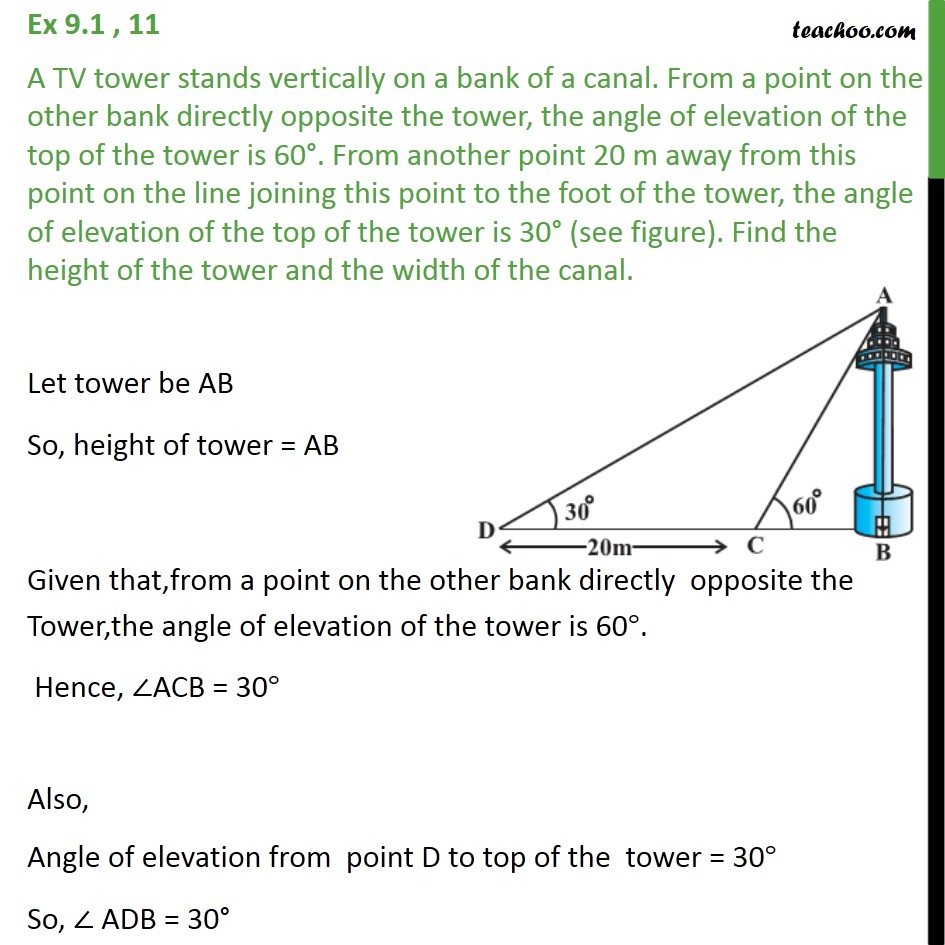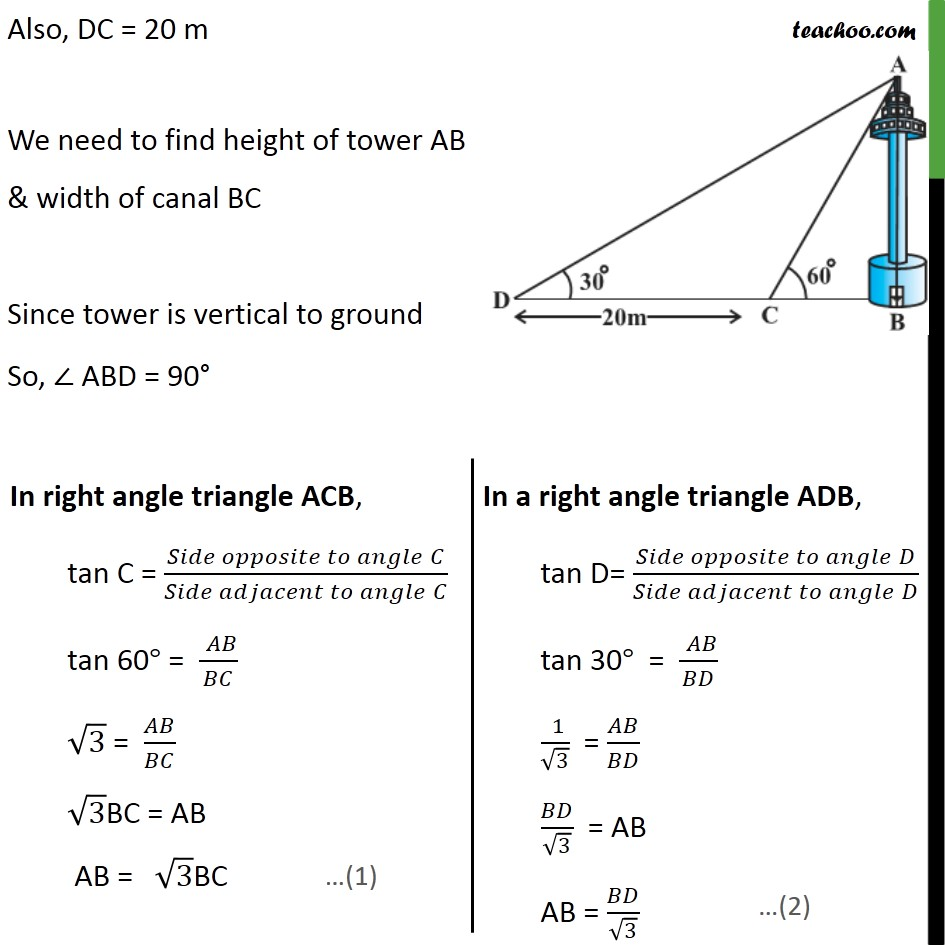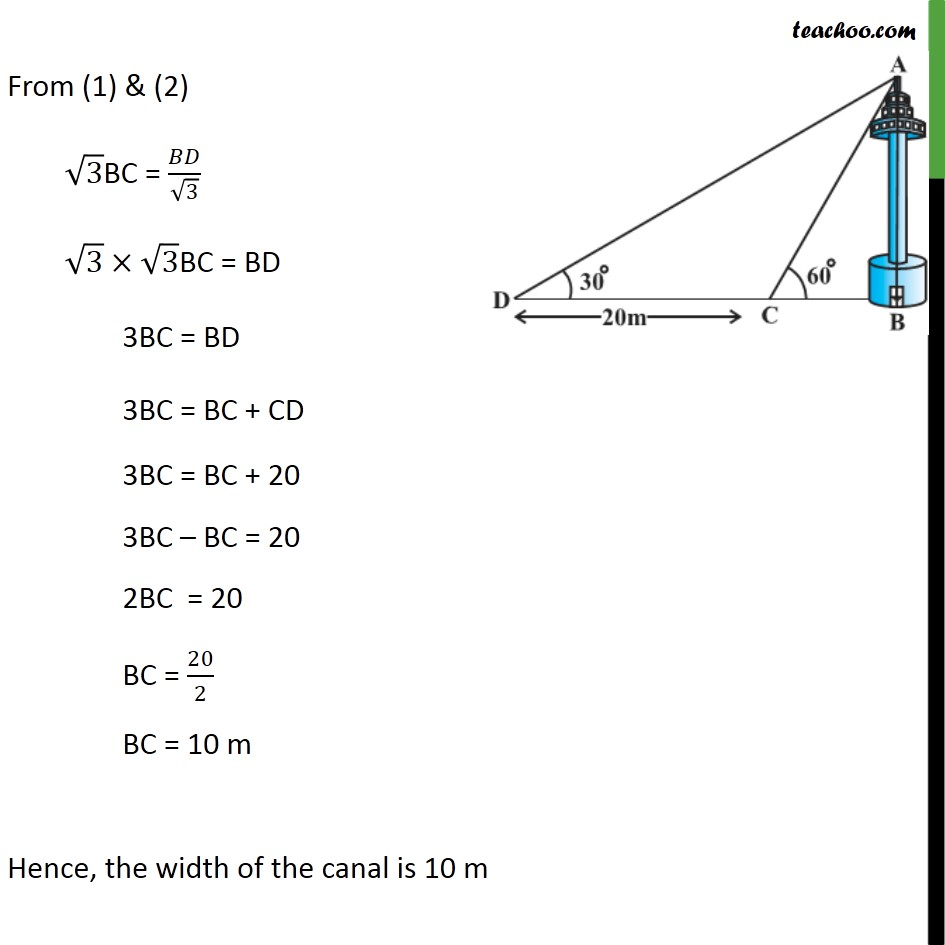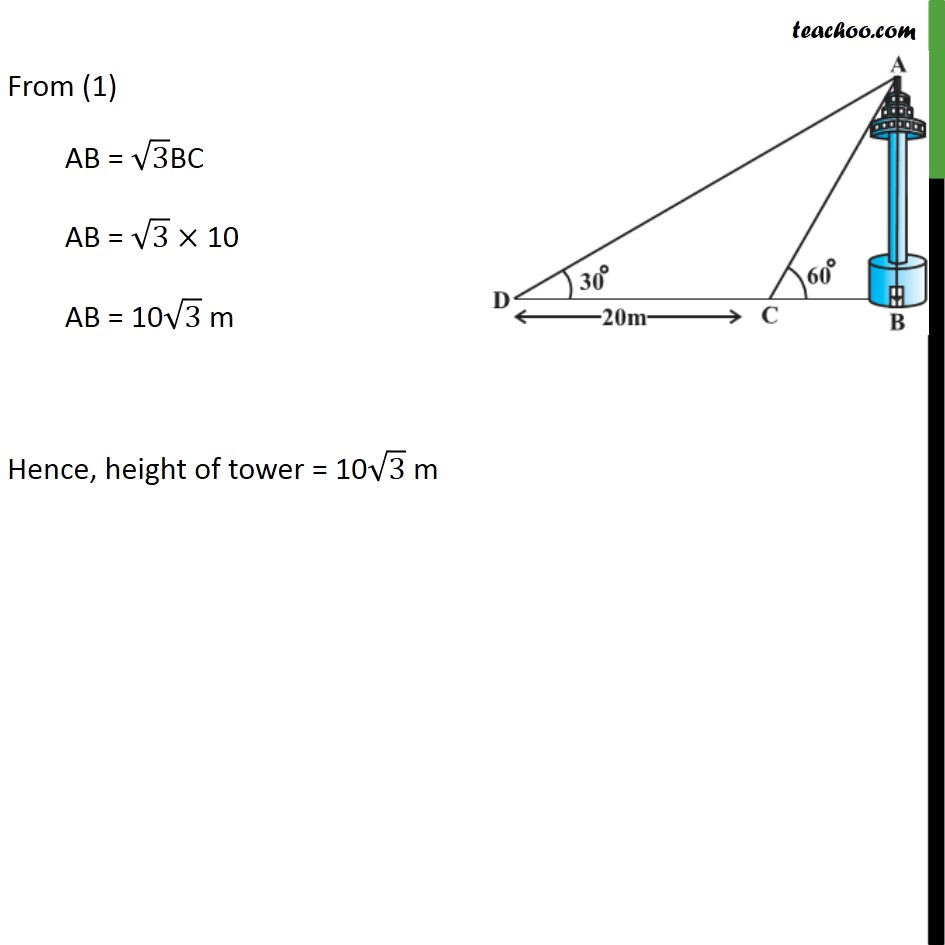1. Chapter 9 Class 10 Some Applications of Trigonometry (Term 2)
2. Concept wise
3. Questions easy to difficult

Transcript

Ex 9.1 , 11 A TV tower stands vertically on a bank of a canal. From a point on the other bank directly opposite the tower, the angle of elevation of the top of the tower is 60 . From another point 20 m away from this point on the line joining this point to the foot of the tower, the angle of elevation of the top of the tower is 30 (see figure). Find the height of the tower and the width of the canal. Let tower be AB So, height of tower = AB Given that,from a point on the other bank directly opposite the Tower,the angle of elevation of the tower is 60 . Hence, ACB = 30 Also, Angle of elevation from point D to top of the tower = 30 So, ADB = 30 Also, DC = 20 m We need to find height of tower AB & width of canal BC Since tower is vertical to ground So, ABD = 90 From (1) & (2) 3BC = / 3 3 3BC = BD 3BC = BD 3BC = BC + CD 3BC = BC + 20 3BC BC = 20 2BC = 20 BC = 20/2 BC = 10 m Hence, the width of the canal is 10 m From (1) AB = 3BC AB = 3 10 AB = 10 3 m Hence, height of tower = 10 3 m